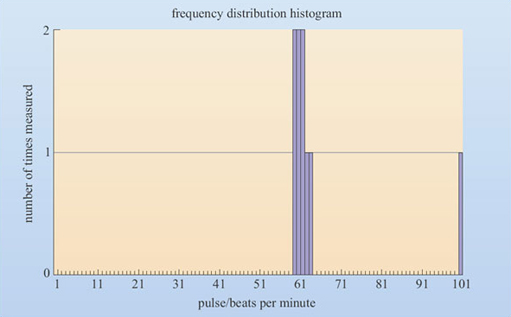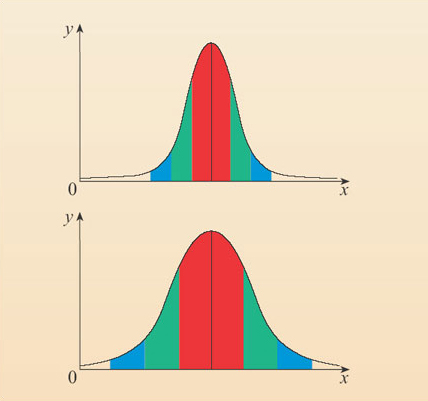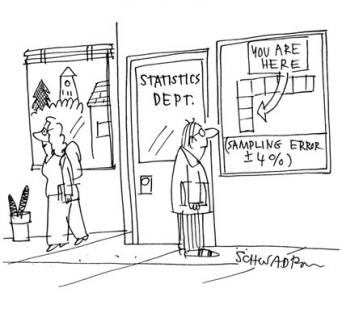Using numbers and handling data

Start this free course now. Just create an account and sign in. Enrol and complete the course for a free statement of participation or digital badge if available.

Free course

# 3.8 Descriptive statistics

## 3.8.1 Standard deviation: finding how reproducible a series of measurements are

Even if we know the maximum and minimum and middle values in a group of numbers, we still don't have a clear idea about the distribution of values within that range: are most of the values all bunched up at one end or spread evenly across the results?

For instance, if I count my pulse rate on the hour every hour, nine times over the course of a day, I might get the following values for the number of beats per minute (bpm): 61, 59, 60, 62, 60, 100, 59, 63, 61. The average result is 65 bpm and range of values is 59-100 bpm. From looking solely at the range you might get the impression that my heart rate fluctuates wildly throughout the day. In fact, my heart rate is remarkably constant, and the value of 100 bpm was a reading, taken after running up the stairs just before 14:00.

The way to find out whether a series of measurements are all tightly grouped together or are spread out more evenly is to make a graph that shows how often a particular value was recorded. This type of graph is called a frequency distribution, because it shows how frequently particular values were recorded.

For instance, in the list of my pulse rate measurements from above:

• 62, 63, and 100 bpm were recorded once

• 59, 60 and 61 bpm were recorded twice.

These data have been plotted on the bar graph shown in Figure 14.Figure 14 A frequency distribution histogram showing how often my pulse rate was measured to be a particular value

With enough measurements, this type of graph eventually resembles a bell-shape, often called a Gaussian curve or a normal distribution, where the most common value is at the top of the curve and there's a spread of less and less common results (some larger and some smaller) on either side. For example if I'd continued to make pulse rate measurements, I would soon have found that my measurement of 100 bpm was a one-off and in fact, most of the measurements were centred around 65 bpm.

Where results are very regularly reproduced and don't deviate much from the mean value (high precision), the bell-shaped curve is steep and narrow (like the top graph in Figure 15) and this indicates a small standard deviation from the mean value (as suggested by the condensed spread of values on the x-axis). In contrast, when the results are more variable (low precision), the bell-shaped curve is relatively spread and flat like the bottom graph in Figure 15, and this indicates a large standard deviation from the mean value.Figure 15 A normal frequency distribution looks like a bell-shaped curve. Top: data showing a small standard deviation from the mean value. Bottom: data showing a large standard deviation from the mean value

The exact value of the standard deviation for a group of numbers is calculated using a complex equation that you are not required to know. Suffice to say that in my pulse rate data above, the mean value is 65 bpm and the standard deviation is 13.2 bpm. Because the standard deviation indicates the spread of data both greater and less than the mean value, it is shown with a 'plus or minus' symbol. Thus, the mean value with the standard deviation is 65 ± 13.2 bpm.

About 68% of all the results occur within one standard deviation of the mean value on the horizontal, x-axis, and this figure is represented by the red areas on both of the graphs in Figure 15). About 95% of the results lie within two standard deviations from the mean (the red plus the green areas on these two graphs), and about 99% of the results lie within three standard deviations of the mean value (the red, green and blue areas).This information can be used to find out if measurements are unusual or not. For instance, we know that 95% of the measurements should be within 2 standard deviations of the mean value, meaning that only 5% of the results will fall outside of two standard deviations. Because the graph is symmetrical, this 5% includes results that are both larger and smaller than the mean value. If we are only interested in results larger than the mean value, then we can see that only 5 ÷ 2 = 2.5% of results occur outside of the green area to the right of the graph. i.e. only 2.5% of the results would be expected to be more than 2 standard deviations greater than the mean value.

In my pulse rate data, one standard deviation was 13.2 bpm and the mean value was 65 bpm. Therefore a pulse rate two standard deviations larger than the mean would be (13.2 × 2) + 65 = 91.4 bpm. As such, I would expect any pulse rate of 91.4 bpm or above to occur less than 2.5% of the time. If today I measured my pulse rate on 5 occasions and it was above 91 bpm on one occasion then that could happen by chance, but if it was this high on subsequent measurements then I should become increasingly worried, since 2 out of the 5 measurements made (i.e. 40%) were above 91 bpm.

S110_1